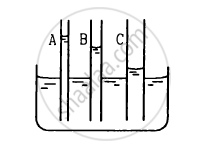Department of Pre-University Education, KarnatakaPUC Karnataka Science Class 11
Advertisement Remove all ads

# The Capillaries Shown in Figure Have Inner Radii 0.5 Mm, 1.0 Mm and 1.5 Mm Respectively. the Liquid in the Beaker is Water. Find the Heights of Water Level in the Capillaries. - Physics

Answer in Brief

The capillaries shown in figure have inner radii 0.5 mm, 1.0 mm and 1.5 mm respectively. The liquid in the beaker is water. Find the heights of water level in the capillaries. The surface tension of water is 7.5 × 10−2 N m−1Advertisement Remove all ads

#### Solution

Given:
Surface tension of water T = 7.5 × 10−2 N/m
Taking cos θ = 1:
Radius of capillary A (rA) = 0.5 mm = 0.5 × 10−3 m

Height of water level in capillary A:

$\text{h}_\text{A } = \frac{2\text{T} \cos \theta}{\text{r}_\text{A} \rho \text{ g}}$

$= \frac{2 \times 7 . 5 \times {10}^{- 2}}{0 . 5 \times {10}^{- 3} \times 1000 \times 10}$

$= 3 \times {10}^{- 2}\text{ m = 3 cm}$

Radius of capillary B (rB) = 1 mm = 1 × 10−3 m

Height of water level in capillary B:

$\text{h}_\text{B} = \frac{2\text{T}\cos \theta}{\text{r}_\text{B} \rho \text{ g}}$

$= \frac{2 \times 7 . 5 \times {10}^{- 2}}{1 \times {10}^{- 3} \times {10}^3 \times 10}$

$= 15 \times {10}^{- 3} \text{ m = 1 . 5 cm }$

Radius of capillary C (rC) = 1.5 mm = 1.5 × 10−3 m
Height of water level in capillary C:

$\text{h}_\text{C} = \frac{2\text{T} \cos \theta}{\text{r}_\text{ C} \rho \text{ g}}$

$= \frac{2 \times 7 . 5 \times {10}^{- 2}}{1 . 5 \times {10}^{- 3} \times {10}^3 \times 10}$

$= \frac{15}{1 . 5} \times {10}^{- 3} \text{ m = 1 cm}$

Is there an error in this question or solution?
Advertisement Remove all ads

#### APPEARS IN

HC Verma Class 11, 12 Concepts of Physics 1
Chapter 14 Some Mechanical Properties of Matter
Q 19 | Page 301
Advertisement Remove all ads

#### Video TutorialsVIEW ALL 

Advertisement Remove all ads
Share
Notifications

View all notifications

Forgot password?